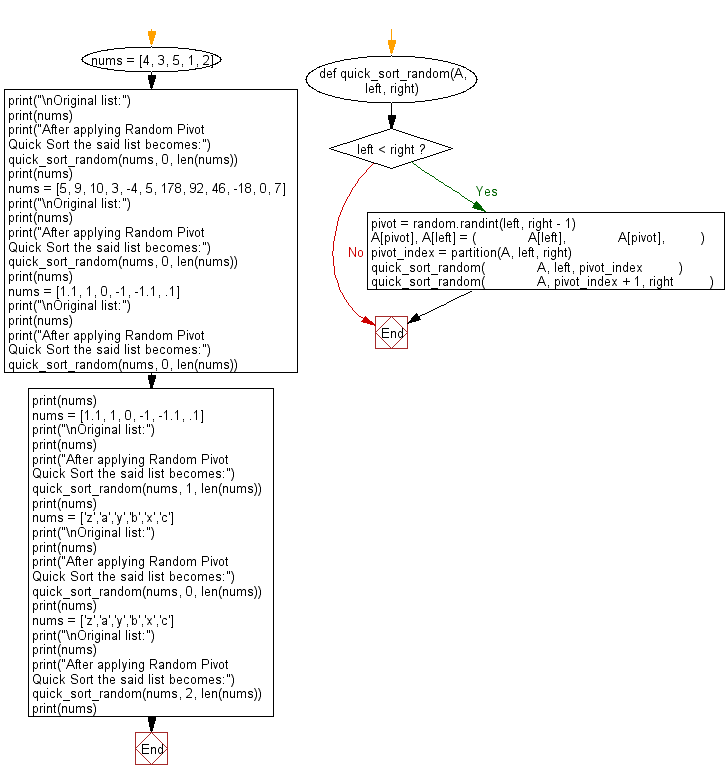﻿ Python: Sort unsorted numbers using Random Pivot Quick Sort - w3resource# Python: Sort unsorted numbers using Random Pivot Quick Sort

## Python Search and Sorting : Exercise-31 with Solution

Write a Python program to sort unsorted numbers using Random Pivot Quick Sort. Picks the random index as the pivot.

Sample Solution:

Python Code:

``````#Ref.https://bit.ly/3pl5kyn
import random

def partition(A, left_index, right_index):
pivot = A[left_index]
i = left_index + 1
for j in range(left_index + 1, right_index):
if A[j] < pivot:
A[j], A[i] = A[i], A[j]
i += 1
A[left_index], A[i - 1] = A[i - 1], A[left_index]
return i - 1
def quick_sort_random(A, left, right):
if left < right:
pivot = random.randint(left, right - 1)
A[pivot], A[left] = (
A[left],
A[pivot],
)  # switches the pivot with the left most bound
pivot_index = partition(A, left, right)
quick_sort_random(
A, left, pivot_index
)  # recursive quicksort to the left of the pivot point
quick_sort_random(
A, pivot_index + 1, right
)  # recursive quicksort to the right of the pivot point
nums = [4, 3, 5, 1, 2]
print("\nOriginal list:")
print(nums)
print("After applying Random Pivot Quick Sort the said list becomes:")
quick_sort_random(nums, 0, len(nums))
print(nums)
nums = [5, 9, 10, 3, -4, 5, 178, 92, 46, -18, 0, 7]
print("\nOriginal list:")
print(nums)
print("After applying Random Pivot Quick Sort the said list becomes:")
quick_sort_random(nums, 0, len(nums))
print(nums)
nums = [1.1, 1, 0, -1, -1.1, .1]
print("\nOriginal list:")
print(nums)
print("After applying Random Pivot Quick Sort the said list becomes:")
quick_sort_random(nums, 0, len(nums))
print(nums)
nums = [1.1, 1, 0, -1, -1.1, .1]
print("\nOriginal list:")
print(nums)
print("After applying Random Pivot Quick Sort the said list becomes:")
quick_sort_random(nums, 1, len(nums))
print(nums)
nums = ['z','a','y','b','x','c']
print("\nOriginal list:")
print(nums)
print("After applying Random Pivot Quick Sort the said list becomes:")
quick_sort_random(nums, 0, len(nums))
print(nums)
nums = ['z','a','y','b','x','c']
print("\nOriginal list:")
print(nums)
print("After applying Random Pivot Quick Sort the said list becomes:")
quick_sort_random(nums, 2, len(nums))
print(nums)
```
```

Sample Output:

```Original list:
[4, 3, 5, 1, 2]
After applying Random Pivot Quick Sort the said list becomes:
[1, 2, 3, 4, 5]

Original list:
[5, 9, 10, 3, -4, 5, 178, 92, 46, -18, 0, 7]
After applying Random Pivot Quick Sort the said list becomes:
[-18, -4, 0, 3, 5, 5, 7, 9, 10, 46, 92, 178]

Original list:
[1.1, 1, 0, -1, -1.1, 0.1]
After applying Random Pivot Quick Sort the said list becomes:
[-1.1, -1, 0, 0.1, 1, 1.1]

Original list:
[1.1, 1, 0, -1, -1.1, 0.1]
After applying Random Pivot Quick Sort the said list becomes:
[1.1, -1.1, -1, 0, 0.1, 1]

Original list:
['z', 'a', 'y', 'b', 'x', 'c']
After applying Random Pivot Quick Sort the said list becomes:
['a', 'b', 'c', 'x', 'y', 'z']

Original list:
['z', 'a', 'y', 'b', 'x', 'c']
After applying Random Pivot Quick Sort the said list becomes:
['z', 'a', 'b', 'c', 'x', 'y']
```

Flowchart:Python Code Editor:

What is the difficulty level of this exercise?

Test your Programming skills with w3resource's quiz.

﻿

## Python: Tips of the Day

Use Reversed() In for Loops:

```>>> tasks = ['laundry', 'picking up kids', 'gardening', 'cooking']